> > Applications of Gauss’s Law

# Applications of Gauss’s Law

Now that you have a brief idea of what Gauss law is, let us look at the application of Gauss Law. Does that already look difficult to you? Well, no! We will make it easier for you! It is important to note that we can use Gauss’s Law to solve complex electrostatic problems involving unique symmetries like cylindrical, spherical or planar symmetry. So, contrary to what you thought, the application of Gauss Law can actually make your task easier!

### Suggested VideosElectrostatic ForceGauss's law and properties of Gaussian SurfaceElectric Field Lines and its properties## Application of Gauss Law

There are various applications of Gauss law which we will look at now. Just to start with, we know that there are some cases in which calculation of electric field is quite complex and involves tough integration. We use the Gauss’s Law to simplify evaluation of electric field in an easy way.

Before we learn more about the applications, let us first see how we can apply the law. We must choose a Gaussian surface, such that the evaluation of the electric field becomes easy. One should make use of symmetry to make problems easier. We must also remember that it is not necessary for the Gaussian surface to coincide with the real surface. It can be inside or outside the Gaussian surface.

## Electric Field due to Infinite Wire

Let us consider an infinitely long wire with linear charge density λ and length L. To calculate electric field, we assume a cylindrical Gaussian surface. As the electric field E is radial in direction, the flux through the end of the cylindrical surface will be zero.

This is because the electric field and area vector are perpendicular to each other. As the electric field is perpendicular to every point of the curved surface, we can say that its magnitude will be constant.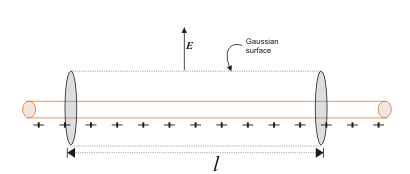The surface area of the curved cylindrical surface is 2πrl. The electric flux through the curve is

E × 2πrl

According to Gauss’s LawYou need to remember that the direction of the electric field is radially outward if linear charge density is positive. On the other hand, it will be radially inward if the linear charge density is negative.

## Electric Field due to Infinite Plate Sheet

Let us consider an infinite plane sheet, with surface charge density σ and cross-sectional area A. The position of the infinite plane sheet is as below: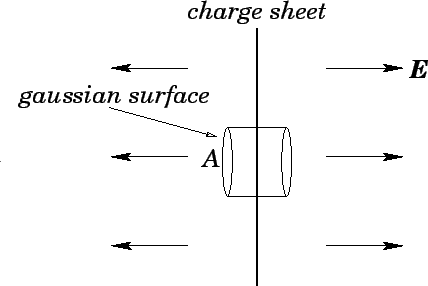The direction of the electric field due to an infinite charge sheet is perpendicular to the plane of the sheet. Let us consider a cylindrical Gaussian surface, whose axis is normal to the plane of the sheet. We can evaluate the electric field E from Gauss’s Law as according to the law: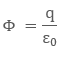Froma continuous charge distribution charge q will be the charge density (σ) times the area (A). Talking about net electric flux, we will consider electric flux only from the two ends of the assumed Gaussian surface. We can attribute it to the fact that the curved surface area and an electric field are normal to each other, thereby producing zero electric flux. So the net electric flux is

Φ = EA – (– EA)

Φ = 2EA

Then, we can write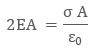The term A cancels out which means electric field due to an infinite plane sheet is independent of cross-sectional area A and equals to: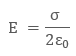## Electric Field due to Thin Spherical Shell

Let us consider a thin spherical shell of surface charge density σ and radius “R”. By observation, we can see that the shell has spherical symmetry. Therefore, we can evaluate the electric field due to the spherical shell in two different positions:

• Electric field outside the spherical shell
• Electric field inside the spherical shell

Let us look at these two cases in greater detail.

###### Electric Field Outside the Spherical Shell

To find electric field outside the spherical shell, we take a point P outside the shell at a distance r from the centre of the spherical shell. By symmetry, we take Gaussian spherical surface with radius r and centre O. The Gaussian surface will pass through P, and experience a constant electric field E all around as all points are equally distanced “r’’ from the centre of the sphere. Then, according to Gauss’s Law: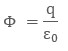The enclosed charge inside the Gaussian surface q will be σ × 4 πR2. The total electric flux through the Gaussian surface will be

Φ = E × 4 πr2

Then by Gauss’s Law, we can write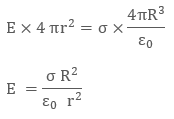Putting the value of surface charge density σ as q/4 πR2, we can rewrite the electric field as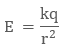In vector form, the electric field is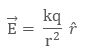where r is the radius vector, depicting the direction of electric field. What we must note here is that if the surface charge density σ is negative, the direction of the electric field will be radially inward.

## Electric Field Inside the Spherical Shell

To evaluate electric field inside the spherical shell, let’s take a point P inside the spherical shell. By symmetry, we again take a spherical Gaussian surface passing through P, centered at O and with radius r. Now according to Gauss’s Law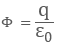The net electric flux will be E × 4 π r2.

## Solved Example for You

Question: Why is there no electric field inside a spherical shell?

Solution: The enclosed charge q will be zero, as we know that surface charge density is dispersed outside the surface, therefore there is no charge inside the spherical shell. Therefore, E = 0

This concludes our discussion on the topic of application of Gauss Law.

Share with friends

## Customize your course in 30 seconds

##### Which class are you in?
5th
6th
7th
8th
9th
10th
11th
12th
Get ready for all-new Live Classes!
Now learn Live with India's best teachers. Join courses with the best schedule and enjoy fun and interactive classes.Ashhar Firdausi
IIT Roorkee
BiologyDr. Nazma Shaik
VTU
ChemistryGaurav Tiwari
APJAKTU
Physics
Get Started

## Browse

##### Electric Charges and FieldsSubscribe
Notify of

## Question Mark?

Have a doubt at 3 am? Our experts are available 24x7. Connect with a tutor instantly and get your concepts cleared in less than 3 steps.